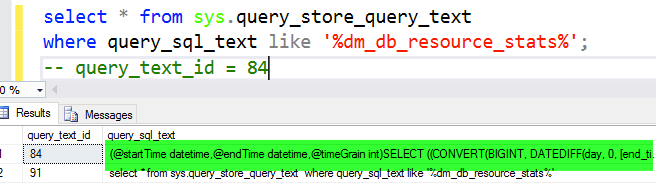# Calculating DTU in Azure SQL Database

A few months ago, I posted a question over on ask.sqlservercentral.com.  In a nutshell, it was how do you measure DTU? How could you pull the data shown in the Azure Portal graphs with T-SQL?  No one answered, so this motivated me get off my butt and answer my question.  In doing so, I wanted to share the results with all my readers as well.

I started thinking like a detective.  If I was DTU how would I want to be found with T-SQL?

Knowing, that both “sys.dm_db_resource_stats” and “sys.resource_stats” holds data that is used to calculate DTU. I decided to leverage Query Store on an Azure SQL Database to see if I could quickly see how DTU is calculated. Behold, I was right.

## Query Store## The Secret Sauce

The whole query is below. Right now, let’s just focus on the secret sauce. The secret sauce is how DTU percentage gets calculated.  In a nutshell, the maximum of CPU, Data IO, Log Write Percent determine your DTU percentage.  What does this mean to you? Your max consumer limits you. So, you can be using 1% of your IO but still be slowed down because CPU could be your max consumer resource.

`(SELECT MAX(v) FROM (VALUES (avg_cpu_percent), (avg_data_io_percent), (avg_log_write_percent)) AS value(v)), 0) as dtu_consumption_percent`

DTU Calculating Query

```SELECT ((CONVERT(BIGINT, DATEDIFF(day, 0, [end_time])) * 24 * 3600 + DATEDIFF(second, DATEADD(day, DATEDIFF(day, 0, [end_time]), 0), [end_time])) / @timeGrain) * @timeGrain as start_time_interval
, MAX(cpu_percent) as cpu_percent
, MAX(log_write_percent) as log_write_percent
, MAX(memory_usage_percent) as memory_usage_percent
, MAX(xtp_storage_percent) as xtp_storage_percent
, MAX(dtu_consumption_percent) as dtu_consumption_percent
, MAX(workers_percent) as workers_percent
, MAX(sessions_percent) as sessions_percent
, MAX(dtu_limit) as dtu_limit
, MAX(dtu_used) as dtu_used
FROM
(SELECT
end_time
, ISNULL(avg_cpu_percent, 0) as cpu_percent
, ISNULL(avg_log_write_percent, 0) as log_write_percent
, ISNULL(avg_memory_usage_percent, 0) as [memory_usage_percent]
, ISNULL(xtp_storage_percent, 0) as xtp_storage_percent
, ISNULL((SELECT MAX(v) FROM (VALUES (avg_cpu_percent), (avg_data_io_percent), (avg_log_write_percent)) AS value(v)), 0) as dtu_consumption_percent
, ISNULL(max_worker_percent, 0) as workers_percent
, ISNULL(max_session_percent, 0) as sessions_percent
, ISNULL(dtu_limit, 0) as dtu_limit
, ISNULL(dtu_limit, 0) * ISNULL((SELECT MAX(v) FROM (VALUES (avg_cpu_percent), (avg_data_io_percent), (avg_log_write_percent)) AS value(v)), 0) / 100.0 as dtu_used
FROM sys.dm_db_resource_stats
WHERE [end_time] >= @startTime AND [end_time] <= @endTime
) t
GROUP BY ((CONVERT(BIGINT, DATEDIFF(day, 0, [end_time])) * 24 * 3600 + DATEDIFF(second, DATEADD(day, DATEDIFF(day, 0, [end_time]), 0), [end_time])) / @timeGrain) * @timeGrain

```

## A Future Blog Post

Now that we can calculate DTU we could trend this data and automatically make changes like moving up and down from the current performance tier.  I would love to wrap this into an Azure SQL Database Alert but a process in PowerShell might be a great starting point.

John Sterrett is a Microsoft Data Platform MVP and a Group Principal for Procure SQL. If you need any help with your on-premise or cloud SQL Server databases, he would love to chat with you. You can contact him directly at john AT ProcureSQL dot com or here.

### 5 responses to “Calculating DTU in Azure SQL Database”

This site uses Akismet to reduce spam. Learn how your comment data is processed.